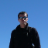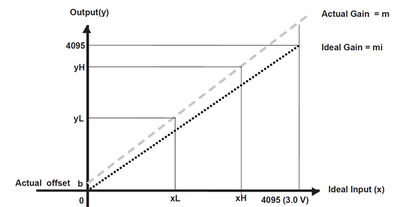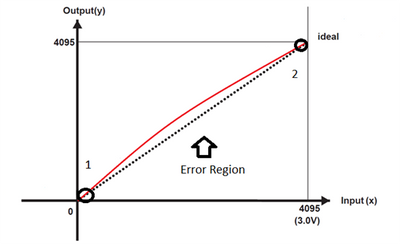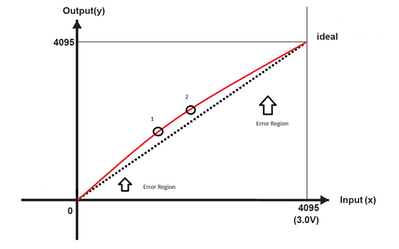## S32K144 Content of the ADC TUE

cancel
Showing results for
Did you mean:

## S32K144 Content of the ADC TUE

275 ViewsContributor I
We would like to apply the S32K144 that is required real 12bit ADC resolution. But unfortunately, S32K144 have the maximum TUE of 8LSB. This means, if we apply the this maximum value, then I expect the same accuracy with 10bit ADC.( 8LSB = 3bit. This means it is same with the 10bit ADC of TUE 0.5LSB.) Therefore we would like to compensate the offset error by the our calibration process. However, we don' know the ration of "Offset error" in the TUE. (Datasheet description "Represents total static error, which includes offset and full scale error.") Please teach us the TUE value when subtract the offset error. Regards,.
5 Replies
250 ViewsNXP TechSupport

Hi Daisaku,

I have asked if we have anything else to provide. I will let you know once I receive some feedback.

Regards,

Lukas

232 ViewsContributor I

Can you have something feedback for my question?

Regards,.

223 ViewsNXP TechSupport

Hi,

I just got the feedback, very detailed indeed. See please below.

***

It is hard to provide a value for the OFFSET error because it could change depending on external factors like EMC or thermal issues. The temperature of the system can have a major influence on ADC accuracy, mainly causing offset error drift and gain error drift.

However the customer could calculate the OFFSET error, that is  present in the particular system/app, let me introduce how perform such calculi:

ADC Offset error is defined as the deviation between the first ideal code transition and the first actual code transition. The first ideal code transition takes place at 0.5 LSB. If the output code is greater than zero when the input voltage is less than 0.5 LSB, the ADC has a positive offset error. ADC has a negative offset error if the first output code transition occurs when the input voltage is greater than 0.5 LSB. Both positive and negative offset errors limit the available range of the ADC. A large positive offset error causes the ADC to saturate before the input voltage reaches maximum. A large negative offset error results in zero ADC output code for small input voltages.

ADC Gain error is defined as the deviation of the midpoint of the last step of the ideal ADC transfer from the midpoint of the last step of the actual ADC, after the offset error is compensated. If the transfer function of the actual ADC results in ADC saturation before the input voltage reaches maximum, a positive gain error is produced. If the transfer function of the actual ADC is such that the ADC does not reach full-scale value when the input voltage is at maximum, a negative gain error is produced. Gain error can also be represented as the full-scale error minus the offset error.

Gain slope for the transfer function can be easily calculated with a two point calibration (rise/run). If you think of the slope of a line with a X-Y graph it is simply Y= mX + b, where in the case of the ADC the analog input voltage value is X, the gain error slope is "m" and the offset error is "b". In the figure below you can visualize the transfer function for a two point calibration.For calibration, it is required to take two ADC measurements at different temperatures and calculate the offsets based off of those two points based in this 2 point calibration method

For the offset relative to what the ADC is seeing from a shorted input case. If the ADC is set to have a zero offset (0 input results in 0 output), then the temperature can be above or below the expected due to gain or linearity errors. Then it is also important to take that behavior in account.

An important consideration for the  last method is to consider just as a broad approach for ADC calibration calculi. It is ok if the measurement remains perfectly linear, but let's visualize (in the next two figures) what happens with a two point measurements technique, if the response of the System/Application, everything together, is non-linear

the next figure would be similar to a system calibration where you have one point with a 0 or the shorted input case, and the other is with a full-scale input case. As you can see you have error in the middle.In that other figure you will see what can happen if the  calibrate is performed in the middle.Now you have error in the measurement on either side of the calibrated region. What you are left with is to calibrate in more regions to create a piece-wise linear correction .The more pieces you have, the closer it represents the actual curve (linear regression). Obviously there is a practical limit to this.

In part, the explanation of why it is not provided the offset error data as part of the DS of the component, but only the ADC TUE.

Hope this helps

Regards,

Lukas

205 ViewsContributor I

Thank you very much for your support. At first, I apologize that I can' understand your comment well. But I would like to understand your explanation by below.

So our circuit will use the 2.35V to 2.65V voltage range only. And we will compensate the offset error by "single" point calibration when assembly line. (Will not perform the temperature calibration.) Therefore we believe, we can compensate the "almost" offset error by this calibration process due to narrow voltage range (0.3V).

In that case, ADC TUE remain the "gain error" and ”part of offset error”. In this case can we consider the TUE of our products less than 6 LSB(at range = +/- 3LSB)?

Regards,.

191 ViewsNXP TechSupport

Hi,

Yes, if you have such narrow operating range, I believe you can compensate the error significantly. However, I can’t tell you the number, I’m afraid it’s up to you to characterize such solution.

Regards,

Lukas